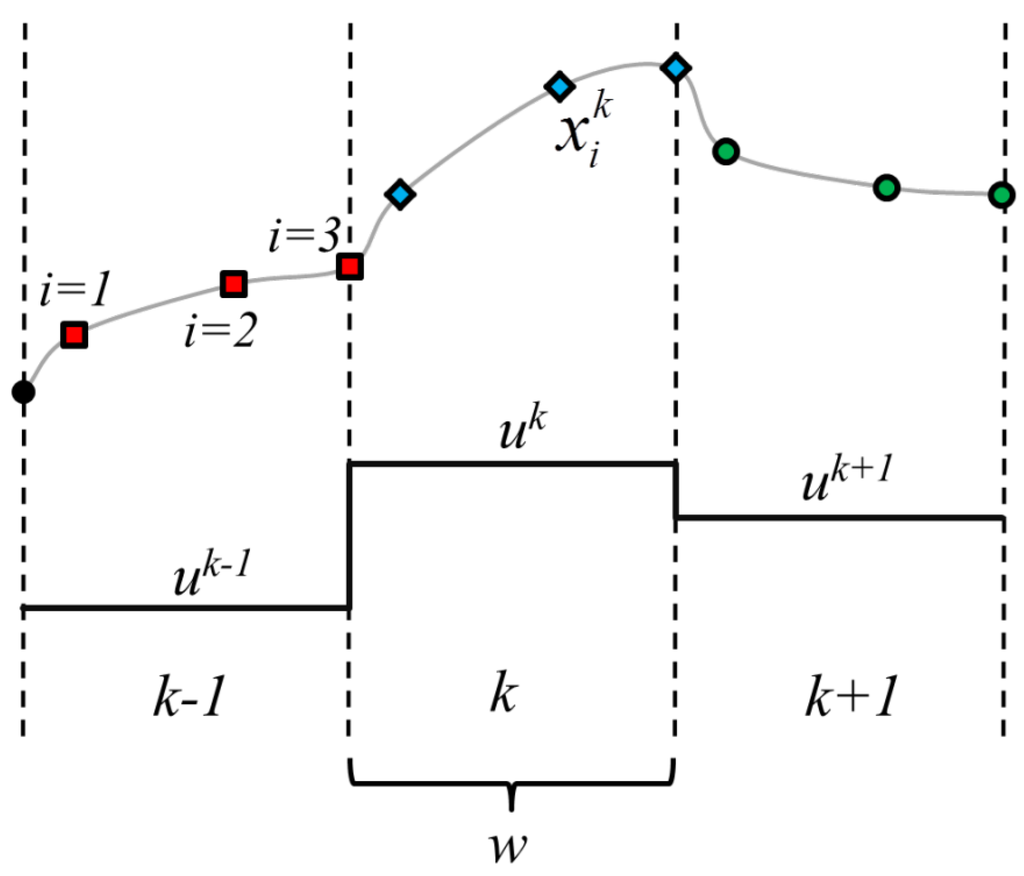Previous Article in Journal
Surrogate Models for Online Monitoring and Process Troubleshooting of NBR Emulsion Copolymerization

Metrics 0

## Export Article

Open AccessArticle
Processes 2016, 4(1), 7; https://doi.org/10.3390/pr4010007

# A Continuous Formulation for Logical Decisions in Differential Algebraic Systems using Mathematical Programs with Complementarity Constraints

1
Department of Chemical Engineering, The University of Utah, Salt Lake City, UT 84112, USA
2
Department of Chemical Engineering, Provo, Brigham Young University, UT 84602, USA
3
McKetta Department of Chemical Engineering, The University of Texas at Austin, Austin, TX 78705, USA
*
Author to whom correspondence should be addressed.
Academic Editor: Michael Henson
Received: 1 January 2016 / Accepted: 11 March 2016 / Published: 21 March 2016
|   PDF [974 KB, uploaded 21 March 2016]   |

# Abstract

This work presents a methodology to represent logical decisions in differential algebraic equation simulation and constrained optimization problems using a set of continuous algebraic equations. The formulations may be used when state variables trigger a change in process dynamics, and introduces a pseudo-binary decision variable, which is continuous, but should only have valid solutions at values of either zero or one within a finite time horizon. This formulation enables dynamic optimization problems with logical disjunctions to be solved by simultaneous solution methods without using methods such as mixed integer programming. Several case studies are given to illustrate the value of this methodology including nonlinear model predictive control of a chemical reactor using a surge tank with overflow to buffer disturbances in feed flow rate. Although this work contains novel methodologies for solving dynamic algebraic equation (DAE) constrained problems where the system may experience an abrupt change in dynamics that may otherwise require a conditional statement, there remain substantial limitations to this methodology, including a limited domain where problems may converge and the possibility for ill-conditioning. Although the problems presented use only continuous algebraic equations, the formulation has inherent non-smoothness. Hence, these problems must be solved with care and only in select circumstances, such as in simulation or situations when the solution is expected to be near the solver’s initial point. View Full-Text
FiguresFigure 1

This is an open access article distributed under the Creative Commons Attribution License which permits unrestricted use, distribution, and reproduction in any medium, provided the original work is properly cited (CC BY 4.0).MDPI and ACS Style

Powell, K.M.; Eaton, A.N.; Hedengren, J.D.; Edgar, T.F. A Continuous Formulation for Logical Decisions in Differential Algebraic Systems using Mathematical Programs with Complementarity Constraints. Processes 2016, 4, 7.

Note that from the first issue of 2016, MDPI journals use article numbers instead of page numbers. See further details here.

1